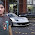Sunday, January 23, 2011

You are provided with four possible operations that can be done on the editor(each operation requires 1 keyboard hit)

1. A
2. Ctrl+A
3. Ctrl+C
4. Ctrl+V
Now you can hit the keyboard N times and you need to find the maximum number of A's that can be printed. Also print the sequence of keyboard hits.

Approach:

Its trivial to have the observation for n <= 6, the max number of A's = n
How about n = 7? If you computed the answer as 8 or 9, open an editor and type the sequence and validate the answer. This is a common gotcha thinking that Ctrl + A, Ctrl + C, Ctrl + V would double the characters but it doesn't :). Infact the characters remains intact while the next Ctrl + V would infact copy the same sequence.

Further thoughts, can you arrive at an optimal number which you can go ahead and copy over and over, or you need a calculated strategy where you refresh your clipboard with current contents. Once you are able to identify this, you should be able to arrive at a proper formative answer

1.It depends upon n
If (n%3==0)
max A= 3 X pow(2,(n-3)/3)
If (n%3==1)
max A = 4 X pow(2,(n-4)/3)
If (n%3==2)
max A = 5 X pow(2,(n-5)/3)

2.Its becoz we hav to do the max use of copy n paste.
Is it correct ??

3.The above ans is when the key sequence is preserved.
If we can use Ctrl+V two times continuously
den it can increase

4.@navin ... yes the Ctrl + v can be used multiple times continiously....

5.Aviral:
nice blog.
please tell me if it's correct:

int MaxA(int n, int len=0, int copyboard =0)
{
if(n < 0)
return 0;
if(n == 0)
return len;

int a = MaxA(n-1, len+1, copyboard); // appending a single 'A'
int b = (copyboard) ? MaxA(n-1, len+copyboard, copyboard) : 0; // pasting the current copyboard
int c = MaxA(n-3, 2*len, len); // hitting CTRL+A, CTRL+C, CTRL+V
return max(a,max(b,c)); // taking the maximum of all possibilities
}

6.@Or ... your code has an exponential time complexity checking over all the possible combinations... you can do much better...

Also the solution has some bugs... in computing the value of 'c'(try it on your editor to get out of trap :))

Regards

7.only n. after ctrl+v, u need a key to change location which is not allowed.

8.after Ctrl+V by default the cursor location is at the end of text .... have a look at ur text editor

Regards

9.Thanks for sharing this google interview questions. Keep posting dude, your blog is more attractive.

Interview Questions